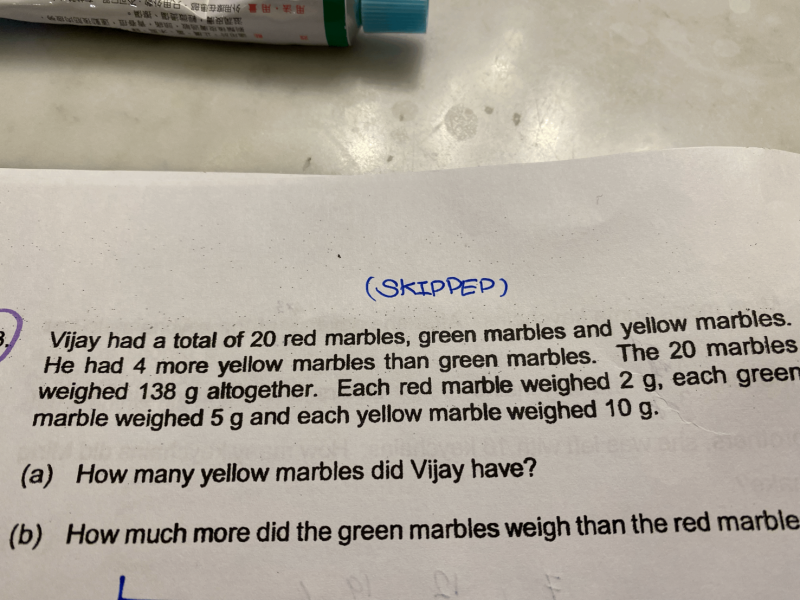# QuestionRed marbles: R, Yellow marbles: Y, Green marbles: G.

R+Y+G=20 and 2R+10Y+5G=138 and Y-G=4                                                            drift boss  1001 tips

solving the system of three equations hidden behind we find:

R= 4, Y= 10, G= 6

0 Replies 1 Like

weight per marble

Yellow    [u]                                                     10g

Green     [u]                                                            5g

Red         [16-2u]    <—- 20-4-2u                       2g

10(u+4) + 5u + 2(16-2u) = 138

10u + 40 + 5u + 32 – 4u = 138

11u = 138-72 = 66

u = 6

(a) u+4 = 6 + 4 = 10

Hence he has 10 Yellow marbles.

(b)  Green  = 6                        weight = 6x 5 = 30

Red = 16 – 2(6) = 4         weight = 4 x 2 = 8

30-8 = 22

Hence the Green marbles weigh 22g more than the Red marbles.

2 Replies 1 Like

Chief,  I agree with you.  The first thing I look was the level too and surprised that it was pitched at P4.   On the other hand, mass is taught at P3.   Also, Money is taught in P3 so the concept of quantity x unit cost is also introduced in the “shopping and budgeting concept”.   Hence, this question is like the application of understanding of Mass and the quantity x unit weight concept?   It’s probably a stretch for most students so the target could be those in the 97th percentile.